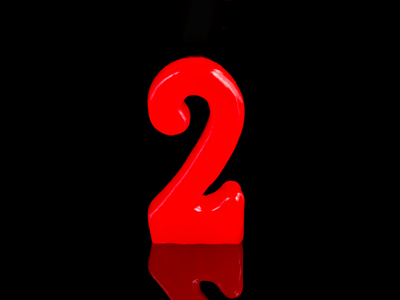All of the numbers in the 8 times table are even - that means that they can all be divided by 2.

# Times Tables (Mixed)

In KS1 Maths and Numeracy children will be exposed to the times tables as early as possible in Year 1. The 1, 2, 5 and 10 times tables are taught first before the 3, 4, 6, 7, 8 and 9 times tables follow. By the end of Year 2 children are often fluent in their times tables up to 10.

This quiz will test children's familiarity with all of their times tables, which will help them in studying maths in Years 1 and 2.

Do you know your times tables? This quiz will test you on them all!

1.
5 x 3 =
10
18
12
15
3 x 5 is the same as 5 + 5 + 5 or 3 + 3 + 3 + 3 + 3
2.
3 x 1 =
3
1
0
6
Don't forget, a number multiplied by one stays the same, so 1 x 1 = 1, 1 x 2 = 2 and 1 x 3 = 3
3.
10 x 0 =
100
1
10
0
Even 10 becomes 0 when it is multiplied by 0
4.
8 x 10 =
72
80
88
64
5.
8 x 3 =
21
24
27
32
6.
4 x 6 =
24
18
20
28
7.
8 x 9 =
75
65
81
72
All of the numbers in the 8 times table are even
8.
6 x 7 =
35
36
42
49
9.
2 x 1 =
0
1
2
4
2 x 1 is the same as 1 + 1
10.
7 x 9 =
63
72
65
56
Author:  Graeme Haw

We use cookies to make your experience of our website better.

To comply with the new e-Privacy directive, we need to ask for your consent -